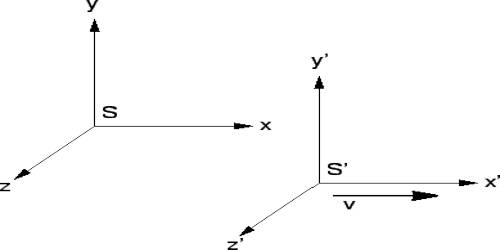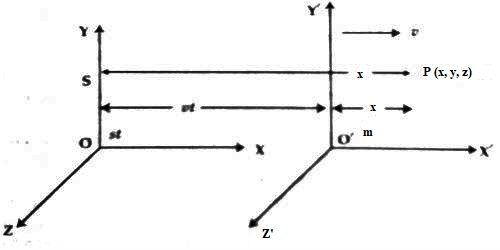# Galilean Transformation

Galilean Transformation

If two events occur simultaneously in two separate frames, then naturally two sets of coordinates will be obtained for the two frames. The equation which is obtained in order to establish the relation between the two sets of coordinates for those two events is called the Galilean transformation equation. In physics, a Galilean transformation is used to transform between the coordinates of two reference frames which differ only by constant relative motion within the constructs of Newtonian physics.

If the two frames are internal frames, then that transformation is also called the Galilean transformation.Fig: Galilean Transformation

Suppose ‘st’ is the stationary frame of reference on the earth’s surface. With respect to it the velocity of the moving m frame along X-axis is v. At time t = 0, if both the origins of the two frames O and Oˊ are at the same place, then after time t = tˊ, point Oˊ will be at a distance ‘vt’ from the point O. If the co-ordinate of the point P is (x, y, x) in the ‘st’ frame, then the coordinates of that point in the frame in will be,

xˊ = x – vt … …. … (1)

Since the frame m is in motion along X-axis, so there will not be any change in Y and Z axes. That means,

yˊ = y … … … (2)

zˊ = z … … … (3)

Time in all frames of reference is the same, so tˊ = t … … … (4)

So, in order to transform an equation of the frame st into the frame m, above equations are to be used. These equations are called Galilean transformation. In this transformation, all the laws of mechanics remain invariant.

By differentiating the equations (1) to (3) the transformation equation for velocities for the frames st and m are obtained.

vxˊ = dxˊ/dt = d/dt (x – vt) = dx/dt – v = vx – v … … … (5)

vyˊ = dyˊ/dt = vy … … … (6)

vzˊ = dzˊ/dt = vz … … … (7)

Equation (5), (6) and (7) are called the transformation equations of velocities. Galilean transformation and velocity transformation are contrary to the postulates of special theory of relativity. How they are contrary that will be discussed now.

According to the first postulate of special relativity laws of physics will remain same in both stationary st and moving m frames of reference. But laws of electromagnetic theories applicable in one frame become different in other frame of reference which is against the first postulate of relativity.

Again, according to the second postulate of special relativity, the speed of light will remain same in stationary st and be moving in frames of reference. But in Galilean transformation, it will be different.

Explanation: Suppose the velocity of light measured along X-axis of the stationary st frame is c. According to equation (5) velocity of light in moving in the frame of reference will be c’ = (c – v), i.e., the velocity of light is dependent on the velocity of the observer which is against the second postulate of the special theory of relativity.